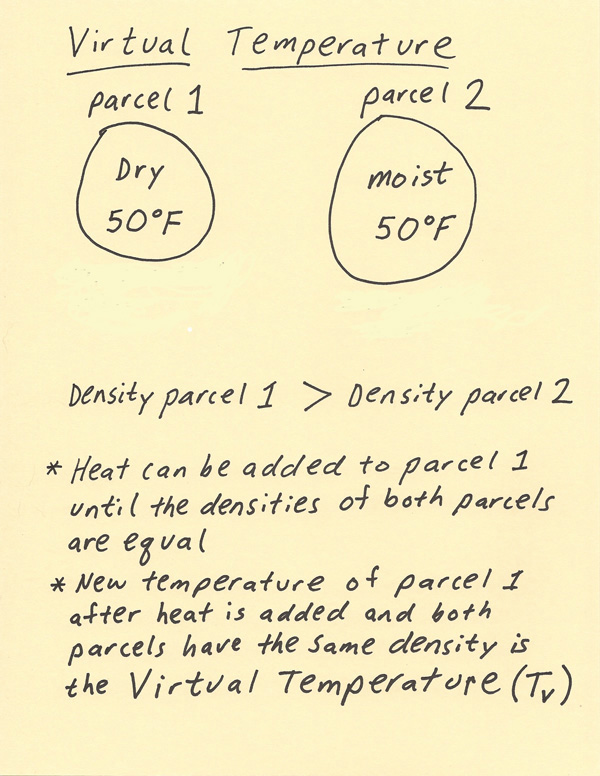[--MAIN HOME--] [--ALL HABYHINTS--] [--FACEBOOK PAGE--]

 HABYTIME MINI LECTURE 37: VIRTUAL TEMPERATURE

METEOROLOGIST JEFF HABY

The virtual temperature is the temperature that a sample of dry air (no moisture) needs in order to match the density of air with moisture. The virtual temperature is typically within 4 degrees C of the actual temperature and is higher than the actual temperature. The virtual temperature is used in meteorological equations to help simplify the equation. Without using the virtual temperature, the equations would have to account in another way for how moisture content changes the density of the air. The use of the virtual temperature is an easy way to account for the moisture in the air such as when using the Hypsometric Equation. The formula for the Virtual Temperature (Tv) is:

Tv = T(1 + 0.61w)
T= temperature in Kelvins
w= mixing ratio in kg of moisture per kg of dry air

In the diagram below there are two parcels of air. Each parcel of air has the same temperature of 50 F. The difference between the parcels is the moisture content. Parcel 1 has no moisture while Parcel 2 does have moisture. Since water vapor (H2O) is lighter than the dominate constituents of air such as diatomic oxygen (O2) and diatomic nitrogen (N2), a parcel of air with moisture will have less mass as compared to a parcel of dry air with the same temperature and volume. Thus, the moist parcel will have a smaller density as compared to the parcel of dry air with the same temperature and volume. In order to make the densities equal, the parcel of dry air needs to be warmed up until it has the same density as the parcel with moisture. Warming the air causes the air to expand. Since the dry air parcel is a higher density than the moist air parcel, the dry air parcel needs to be warmed up in order to decrease its density until it is the same density as the moist parcel. This is why the virtual temperature is a higher temperature than the actual temperature.